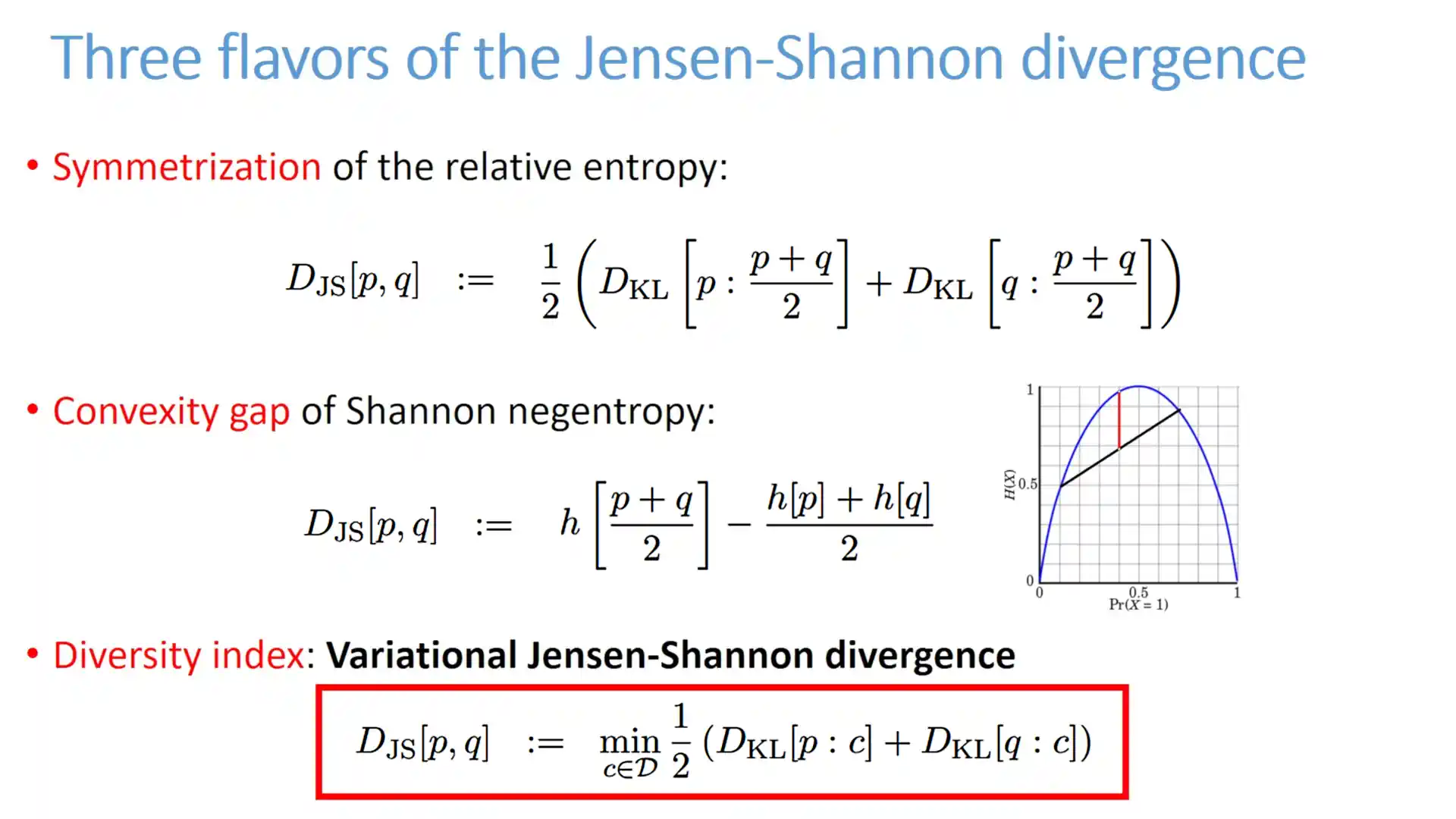# Informations

## Entropies and other measures of surprise

TODO: explain this diagram which I ripped off from Wikipedia.Informations as measure, Venn diagram

Not: what you [hope to get from the newspaperepistemic community. (Although…) Rather: Different types of (formally defined) entropy/information and their disambiguation. The seductive power of the logarithm and convex functions rather like it.

A proven path to publication is to find or reinvent a derived measure based on Shannon information, and apply it to something provocative-sounding. (Qualia! Stock markets! Evolution! Language! The qualia of evolving stock market languages!)

This is purely about the analytic definition given random variables. If you wish to estimate such a quantity empirically, from your experiment, that’s a different problem.

Connected also to functional equations and yes, statistical mechanics, and quantum information physics.

## Shannon Information

Vanilla information, thanks be to Claude Shannon. You have are given a discrete random process of specified parameters. How much can you compress it down to a more parsimonious process? (leaving coding theory aside for the moment.)

Given a random variable $$X$$ taking values $$x \in \mathcal{X}$$ from some discrete alphabet $$\mathcal{X}$$, with probability mass function $$p(x)$$.

$\begin{array}{ccc} H(X) & := & -\sum_{x \in \mathcal{X}} p(x) \log p(x) \\ & \equiv & E( \log 1/p(x) ) \end{array}$

More generally if we have a measure $$P$$ over some Borel space

$H(X)=-\int _{X}\log {\frac {\mathrm {d} P}{\mathrm {d} \mu }}\,dP$

Over at the Functional equations page I note that Tom Leinster has a clever proof of the optimality of Shannon information via functional equations.

One interesting aspect of the proof is where the difficulty lies. Let $$I:\Delta_n \to \mathbb{R}^+$$ be continuous functions satisfying the chain rule; we have to show that $$I$$ is proportional to $$H$$. All the effort and ingenuity goes into showing that $$I$$ is proportional to $$H$$ when restricted to the uniform distributions. In other words, the hard part is to show that there exists a constant $$c$$ such that

$I(1/n, \ldots, 1/n) = c H(1/n, \ldots, 1/n)$

for all $$n \geq 1$$.

Venkatesan Guruswami, Atri Rudra and Madhu Sudan, Essential Coding Theory.

## K-L divergence

Because “Kullback-Leibler divergence” is a lot of syllables for something you use so often, even if usually in sentences like “unlike the K-L divergences”. Or you could call it the “relative entropy”, but that sounds like something to do with my uncle after the seventh round of Christmas drinks.

It is defined between the probability mass functions of two discrete random variables, $$X,Y$$ over the same space, where those probability mass functions are given $$p(x)$$ and $$q(x)$$ respectively.

$\begin{array}{cccc} D(P \parallel Q) & := & -\sum_{x \in \mathcal{X}} p(x) \log p(x) \frac{p(x)}{q(x)} \\ & \equiv & E \log p(x) \frac{p(x)}{q(x)} \end{array}$

More generally, if the random variables have laws, respectively $$P$$ and $$Q$$:

$D_{\operatorname {KL} }(P\|Q)=\int _{\operatorname {supp} P}{\frac {\mathrm {d} P}{\mathrm {d} Q}}\log {\frac {\mathrm {d} P}{\mathrm {d} Q}}\,dQ=\int _{\operatorname {supp} P}\log {\frac {\mathrm {d} P}{\mathrm {d} Q}}\,dP,}$

## Jenson-Shannon divergence

Symmetrized version.## Mutual information

The “informativeness” of one variable given another… Most simply, the K-L divergence between the product distribution and the joint distribution of two random variables. (That is, it vanishes if the two variables are independent).

Now, take $$X$$ and $$Y$$ with joint probability mass distribution $$p_{XY}(x,y)$$ and, for clarity, marginal distributions $$p_X$$ and $$p_Y$$.

Then the mutual information $$I$$ is given

$I(X; Y) = H(X) - H(X|Y)$

Estimating this one has been giving me grief lately, so I’ll be happy when I get to this section and solve it forever. See nonparametric mutual information.

Getting an intuition of what this measure does is handy, so I’ll expound some equivalent definitions that emphasises different characteristics:

$\begin{array}{cccc} I(X; Y) & := & \sum_{x \in \mathcal{X}} \sum_{y \in \mathcal{Y}} p_{XY}(x, y) \log p(x, y) \frac{p_{XY}(x,y)}{p_X(x)p_Y(y)} \\ & = & D( p_{XY} \parallel p_X p_Y) \\ & = & E \log \frac{p_{XY}(x,y)}{p_X(x)p_Y(y)} \end{array}$

More usually we want the Conditional Mutual information.

$I(X;Y|Z)=\int _{\mathcal {Z}}D_{\mathrm {KL} }(P_{(X,Y)|Z}\|P_{X|Z}\otimes P_{Y|Z})dP_{Z}$

See is Chrosopher Olah’s excellent visual explanation.

## Kolmogorov-Sinai entropy

Schreiber says:

If $$I$$ is obtained by coarse graining a continuous system $$X$$ at resolution $$\epsilon$$, the entropy $$HX(\epsilon)$$ and entropy rate $$hX(\epsilon)$$ will depend on the partitioning and in general diverge like $$\log(\epsilon)$$ when $$\epsilon \to 0$$. However, for the special case of a deterministic dynamical system, $$\lim_{\epsilon\to 0} hX (\epsilon) = hKS$$ may exist and is then called the Kolmogorov-Sinai entropy. (For non-Markov systems, also the limit $$k \to \infty$$ needs to be taken.)

That is, it is a special case of the entropy rate for a dynamical system - Cue connection to algorithmic complexity. Also metric entropy?

## Relatives

### Rényi Information

Also, the Hartley measure.

You don’t need to use a logarithm in your information summation. Free energy, something something. (?)

The observation that many of the attractive features of information measures are simply due to the concavity of the logarithm term in the function. So, why not whack another concave function with even more handy features in there? Bam, you are now working on Rényi information. How do you feel?

### Tsallis statistics

Attempting to make information measures “non-extensive”. “q-entropy”. Seems to have made a big splash in Brazil, but less in other countries. Non-extensive measures are an intriguing idea, though. I wonder if it’s parochialism that keeps everyone off Tsallis statistics, or a lack of demonstrated usefulness?

## Estimating information

Wait, you don’t know the exact parameters of your generating process a priori? You need to estimate it from data.